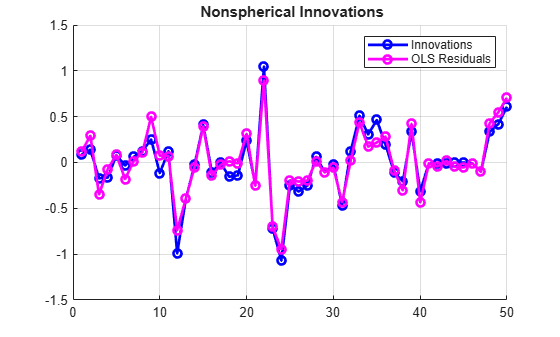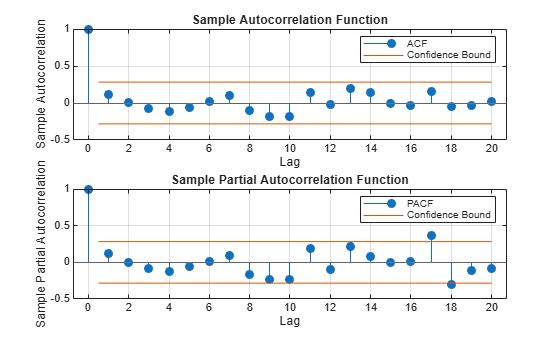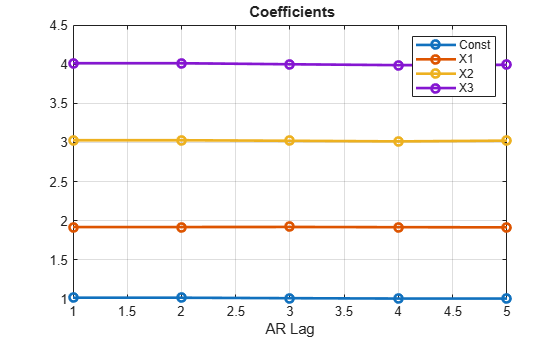Documentation

## Time Series Regression X: Generalized Least Squares and HAC Estimators

This example shows how to estimate multiple linear regression models of time series data in the presence of heteroscedastic or autocorrelated (nonspherical) innovations. It is the tenth in a series of examples on time series regression, following the presentation in previous examples.

### Introduction

Multiple linear regression models are often specified with an innovations process that is known to be either heteroscedastic or autocorrelated (nonspherical). If other regularity conditions of the Classical Linear Model (CLM) continue to hold (see the example on "Linear Models"), ordinary least squares (OLS) estimates of the regression coefficients remain unbiased, consistent, and, if the innovations are normally distributed, asymptotically normal. However, the estimates are no longer efficient, relative to other estimators, and $t$ and $F$ tests are no longer valid, even asymptotically, because the standard formulas for estimator variance become biased. As a result, the significance of the OLS coefficient estimates is distorted (see the example on "Residual Diagnostics").

The usual prescription for such cases is to respecify the model, choosing alternate predictors to minimize nonspherical characteristics in the residuals. However, this is not always practical. Predictors are often selected on the basis of theory, policy, or available data, and alternatives may be limited. Lagged predictors, used to account for autocorrelations, introduce additional problems (see the example on "Lagged Variables and Estimator Bias"). This example explores two approaches that acknowledge the presence of nonsphericality, and revise OLS estimation procedures accordingly.

The first approach is to use heteroscedasticity-and-autocorrelation-consistent (HAC) estimates of OLS standard errors. OLS coefficient estimates are unchanged, but tests of their significance become more reliable. Various types of HAC estimators are implemented by the Econometrics Toolbox function hac.

The second approach modifies the OLS coefficient estimates, by explicitly incorporating information about an innovation covariance matrix of more general form than ${\sigma }^{2}I$. This is known as Generalized Least Squares (GLS), and for a known innovations covariance matrix, of any form, it is implemented by the Statistics and Machine Learning Toolbox™ function lscov. Unfortunately, the form of the innovations covariance matrix is rarely known in practice. The Econometrics toolbox function fgls implements a Feasible Generalized Least Squares (FGLS) procedure which estimates the innovations covariance matrix using specified models, before applying GLS to obtain regression coefficients and their standard errors.

### Nonspherical Innovations

To demonstrate, we simulate a data-generating process (DGP) with known regression coefficients (1, 2, 3, 4), paired with a known nonspherical innovations process. As is typical with econometric models, the innovations include some degree of both heteroscedasticity and autocorrelation. The goal of a regression analysis is to recover the coefficients as accurately as possible from the simulated data.

% Simulate data: numObs = 50; % Number of observations rng(0); % Reset random number generators X = randn(numObs,3); % 3 random predictors % Simulate innovations: var = 0.1; phi = [0.5,0.3]; % Autocorrelation coefficients e = simulate(arima('Constant',0,'AR',phi,'Variance',var),numObs); e = X(:,1).*e; % Heteroscedasticity proportional to first predictor % Simulate response: b = [1;2;3;4]; % Regression coefficients, including intercept y = [ones(numObs,1),X]*b + e; % Store data: DataTable = array2table([X,y],'VariableNames',{'X1','X2','X3','Y'});

The predictors in the simulation are not exogenous to the model, since the innovations are specified as the product of the first predictor and an AR(2) process. This maintains contemporaneous non-correlation between the predictors and the innovations (no linear relations between them), but the variances are correlated.

### OLS Estimates

We first estimate coefficients and standard errors using OLS formulas based on CLM assumptions:

OLSModel = fitlm(DataTable)
OLSModel = Linear regression model: Y ~ 1 + X1 + X2 + X3 Estimated Coefficients: Estimate SE tStat pValue ________ ________ ______ __________ (Intercept) 1.016 0.05289 19.21 1.3187e-23 X1 1.9171 0.041097 46.649 2.1891e-40 X2 3.0239 0.050195 60.243 2.0541e-45 X3 4.022 0.047813 84.12 5.044e-52 Number of observations: 50, Error degrees of freedom: 46 Root Mean Squared Error: 0.359 R-squared: 0.997, Adjusted R-Squared: 0.996 F-statistic vs. constant model: 4.38e+03, p-value = 1.62e-56 

The OLS estimates approximate the coefficients in the DGP, and the $t$ statistics appear to be highly significant.

The residual series, however, displays both the heteroscedasticity and autocorrelation (which, in simulation only, can be compared directly with the innovations):

res = OLSModel.Residuals.Raw; figure hold on plot(e,'bo-','LineWidth',2) plot(res,'mo-','LineWidth',2) hold off legend({'Innovations','OLS Residuals'}) title('{\bf Nonspherical Innovations}') grid on### HAC Estimates

HAC estimators are designed to correct for the bias in the OLS standard error calculation introduced by nonspherical innovations, and so provide a more robust setting for inference regarding the significance of OLS coefficients. An advantage of HAC estimators is that they do not require detailed knowledge of the nature of the heteroscedasticity or autocorrelation in the innovations in order to compute consistent estimates of the standard errors.

HAC estimates using the quadratic spectral (QS) kernel achieve an optimal rate of consistency :

hac(DataTable,'weights','QS','display','full');
Estimator type: HAC Estimation method: QS Bandwidth: 2.9266 Whitening order: 0 Effective sample size: 50 Small sample correction: on Coefficient Estimates: | Coeff SE ------------------------ Const | 1.0160 0.0466 X1 | 1.9171 0.0628 X2 | 3.0239 0.0569 X3 | 4.0220 0.0296 Coefficient Covariances: | Const X1 X2 X3 -------------------------------------------- Const | 0.0022 0.0007 -0.0005 -0.0004 X1 | 0.0007 0.0039 -0.0011 -0.0002 X2 | -0.0005 -0.0011 0.0032 0.0004 X3 | -0.0004 -0.0002 0.0004 0.0009 

The size of the standard errors, and so the reliability of the OLS coefficient estimates, changes relative to the OLS calculation, above. Although positive autocorrelations, typical in economic data, tend to produce downward bias in OLS standard errors, the effect can be obscured in finite samples, and by the presence of heteroscedasticity. Here, some of the standard errors increase in the HAC estimates, and others decrease.

There are many models of heteroscedasticity and autocorrelation built into the hac framework. A thorough analysis of the reliability of coefficient standard errors would involve the use of several models, with different settings for the associated parameters. See, for example, .

 recommends prewhitening HAC estimators to reduce bias. The procedure tends to increase estimator variance and mean-squared error, but can improve confidence interval coverage probabilities and reduce the over-rejection of $t$ statistics. The procedure is implemented via the 'whiten' parameter of hac, but it involves a "nuisance parameter" (the order of a VAR model) which must be investigated for sensitivity:

for order = 0:3 [~,se] = hac(DataTable,'weights','QS','whiten',order,'display','off') end
se = 4×1 0.0466 0.0628 0.0569 0.0296 
se = 4×1 0.0553 0.0801 0.0612 0.0347 
se = 4×1 0.1082 0.1486 0.1795 0.0390 
se = 4×1 0.1153 0.1337 0.1827 0.0361 

The 0-order model bypasses the prewhitening filter, to provide the same results as before. The widening and tightening of the standard error intervals at different whitening orders illustrates the practical difficulties of tuning, and interpreting, the procedure.

### FGLS Estimates

An alternative to HAC estimators are FGLS estimators (also known as Estimated GLS, or EGLS, estimators), for both regression coefficients and their standard errors. These estimators make use of revised formulas which explicitly incorporate the innovations covariance matrix. The difficulty of using FGLS estimators, in practice, is providing an accurate estimate of the covariance. Again, various models are employed, and estimated from the residual series, but numerical sensitivities often provide challenges.

The first step in identifying an appropriate covariance model is to examine the residual series from an initial OLS regression. Analyses of this type are provided in the example on "Residual Diagnostics." Based on the obvious heteroscedasticity in the plot of the raw residuals, above, a diagonal covariance model, such as the 'HC1' option for the 'innovModel' parameter in fgls, is a reasonable choice:

fgls(DataTable,'innovMdl','HC1','display','final');
OLS Estimates: | Coeff SE ------------------------ Const | 1.0160 0.0529 X1 | 1.9171 0.0411 X2 | 3.0239 0.0502 X3 | 4.0220 0.0478 FGLS Estimates: | Coeff SE ------------------------ Const | 1.0117 0.0068 X1 | 1.9166 0.0062 X2 | 3.0256 0.0072 X3 | 4.0170 0.0067 

The coefficient estimates are similar to those for OLS, but the standard errors are significantly reduced.

To consider the effects of autocorrelation in the residuals, and identify an appropriate lag order for an AR model of the covariance, autocorrelation plots are helpful:

figure subplot(2,1,1) autocorr(res) subplot(2,1,2) parcorr(res)The plots show no evidence of significant autocorrelation. As before, the autocorrelation appears to be obscured by the heteroscedasticity. Hypothesis tests, such as the Ljung-Box Q-test, are equally ineffective in discovering the autocorrelation in the DGP. This situation is typical in practice, and points to the difficulty of specifying an accurate model of the innovations covariance.

Autoregressive covariance models use the 'AR' option for the 'innovModel' parameter in fgls. Without evidence of a specific lag order for the model, however, this involves selection of another "nuisance parameter":

numLags = 5; % Consider models with up to this many AR lags. numCoeffs = 4; coeffs = zeros(numLags,numCoeffs); ses = zeros(numLags,numCoeffs); for lag = 1:numLags [coeff,se] = fgls(DataTable,'innovMdl','AR','arLags',lag); coeffs(lag,:) = coeff'; ses(lag,:) = se'; end figure plot(coeffs,'o-','LineWidth',2) set(gca,'XTick',1:numLags) xlabel('AR Lag') legend({'Const','X1','X2','X3'}) title('{\bf Coefficients}') grid onfigure plot(ses,'o-','LineWidth',2) set(gca,'XTick',1:numLags) xlabel('AR Lag') legend({'Const','X1','X2','X3'}) title('{\bf Standard Errors}') grid onThe plots show little effect on the estimates across a range AR model orders, with only the standard error of the intercept estimate changing significantly.

FGLS estimation is often iterated, by recomputing the residuals, and so the covariance estimate, at each step. The asymptotic distributions of FGLS estimators are unchanged after the first iteration, but the effect on finite sample distributions is much less understood. The numIter parameter in the fgls function provides a mechanism for investigating the behavior of iterated FGLS estimates in specific cases:

fgls(DataTable,'numIter',5,'plot',{'coeff','se'});In this case, the default AR(1) model is iterated five times. The estimates converge after just a few iterations.

FGLS estimates are biased but consistent, and asymptotically more efficient than OLS estimates, when the predictors are weakly dependent and strictly exogenous. Without exogeneity of the predictors, however, FGLS is no longer consistent, in general (and so not efficient). For the type of non-exogeneity presented in the simulation, there is no harm to estimator consistency.

FGLS estimators have a long history in econometrics. Early computational methods, like the Cochrane-Orcutt procedure and its variants (Prais-Winsten, Hatanaka, Hildreth-Lu, etc.), used OLS methods to estimate the parameters in the covariance models (typically, AR(1) or AR(2)). Modern FGLS estimators, such as fgls, use the asymptotically more efficient technique of maximum likelihood estimation (MLE) to compute model parameters, but the overall approach is the same.

### Summary

When a regression model is "misspecified" with respect to CLM assumptions, and the residual series exhibits nonspherical behavior, HAC and FGLS estimators can be useful tools in assessing the reliability of model coefficients. As this example demonstrates, neither approach is without its limitations in finite samples. It is useful to remember that FGLS estimators require strictly exogenous regressors, and specific models of the innovations covariance, in order to provide reliable results. HAC estimators demand much less initial diagnostic information, but often provide comparably reduced accuracy. In general, as in most econometric analyses, multiple techniques should be used as part of a more comprehensive review of estimator sensitivities. The hac and fgls interfaces in Econometrics Toolbox provide flexible frameworks for conducting these investigations.

### References

 Andrews, D. W. K. "Heteroskedasticity and Autocorrelation Consistent Covariance Matrix Estimation." Econometrica. v. 59, 1991, pp. 817-858.

 Andrews, D. W. K., and J. C. Monohan. "An Improved Heteroskedasticity and Autocorrelation Consistent Covariance Matrix Estimator." Econometrica. v. 60, 1992, pp. 953-966.

 Box, G. E. P., G. M. Jenkins, and G. C.Reinsel. Time Series Analysis: Forecasting and Control. Englewood Cliffs, NJ: Prentice Hall, 1994.

 Davidson, R. and J.G. MacKinnon. Econometric Theory and Methods. Oxford, UK: Oxford University Press, 2004.

 Greene, W.H. Econometric Analysis. Upper Saddle River, NJ: Pearson Prentice Hall, 2008.

 Hamilton, J. D. Time Series Analysis. Princeton, NJ: Princeton University Press, 1994.

 Judge, G. G., W. E. Griffiths, R. C. Hill, H. Lutkepohl, and T. C. Lee. The Theory and Practice of Econometrics. New York, NY: John Wiley & Sons, Inc., 1985.